# Problem 51715. Iterate the sum of divisors and totient

Cody Problem 46898 deals with the sum of divisors function, denoted by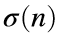, while Cody Problem 656 deals with the totient function, denoted by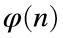. The sum of divisors is straightforward: for example,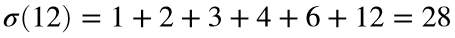. The totient of n counts the numbers less than n that are relatively prime to n. For example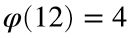because the greatest common divisor of 12 and four numbers (1, 5, 7, 11) is 1.
What happens if you repeatedly apply the two functions, starting with the sum of divisors and alternating? For example, start with 7. Then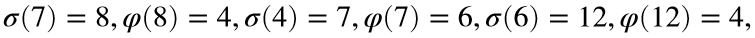etc.
and the pattern 7, 6, 12, 4 will repeat.
Oscillating behavior is plausible because the sum of divisors is always greater than n, and the totient is always smaller than n. Furthermore, because the totient has a minimum value and the sum of divisors has a maximum value, with enough iterations the sequence would have to hit a repeating pattern.
Write a function that takes an initial seed and returns the repeating pattern and the index of the sequence where the pattern begins. With an initial seed of 7, the sequence would be 7, 8, 4, 7, 6, 12, 4, 7, 6, 12,… Therefore, the repeating pattern is [7 6 12 4] and the start index is 3.

### Solution Stats

82.35% Correct | 17.65% Incorrect
Last Solution submitted on May 31, 2023

### Community Treasure Hunt

Find the treasures in MATLAB Central and discover how the community can help you!

Start Hunting!